# Colleges with the lowest SAT scores in Virginia

Top 10 colleges in Virginia with the lowest SAT scores
Looking for the colleges with the lowest SAT scores in Virginia? Well you're in luck! We've compiled a national college database and have created a list of the top 10 universities with the lowest SAT scores in Virginia below. If you are not a good test taker or worried about your test scores, this list is for you. These are the schools whose applicants had the lowest average SAT scores in Virginia, which means that you can get into these colleges with a lower SAT score. We also include each college's ACT scores and acceptance rate so that you can see where you would have the easiest time getting in. Read on to find out more.

## Virginia State University SAT scores

The average SAT score for Virginia State University is 920.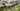The average SAT score of 920 breaks down into:

• SAT math: 450

The average ACT score for Virginia State University is 17 and their acceptance rate is 91%.

## Virginia Union University SAT scores

The average SAT score for Virginia Union University is 930.The average SAT score of 930 breaks down into:

• SAT math: 430

The average ACT score for Virginia Union University is 16 and their acceptance rate is 57.3%.

## Ferrum College SAT scores

The average SAT score for Ferrum College is 940.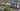The average SAT score of 940 breaks down into:

• SAT math: 470

The average ACT score for Ferrum College is 20 and their acceptance rate is 73.3%.

## Norfolk State University SAT scores

The average SAT score for Norfolk State University is 950.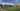The average SAT score of 950 breaks down into:

• SAT math: 470

The average ACT score for Norfolk State University is 18 and their acceptance rate is 67.3%.

## Averett University SAT scores

The average SAT score for Averett University is 995.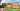The average SAT score of 995 breaks down into:

• SAT math: 500

The average ACT score for Averett University is 19 and their acceptance rate is 61%.

## University of Virginia’s College at Wise SAT scores

The average SAT score for University of Virginia’s College at Wise is 1030.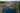The average SAT score of 1030 breaks down into:

• SAT math: 500

The average ACT score for University of Virginia’s College at Wise is 20 and their acceptance rate is 77.5%.

The average SAT score for Radford University is 1041.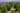The average SAT score of 1041 breaks down into:

• SAT math: 510

The average ACT score for Radford University is 20 and their acceptance rate is 74.6%.

## Longwood University SAT scores

The average SAT score for Longwood University is 1052.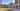The average SAT score of 1052 breaks down into:

• SAT math: 510

The average ACT score for Longwood University is 21 and their acceptance rate is 91.8%.

## Virginia Wesleyan College SAT scores

The average SAT score for Virginia Wesleyan College is 1060.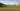The average SAT score of 1060 breaks down into:

• SAT math: 520

The average ACT score for Virginia Wesleyan College is 22 and their acceptance rate is 70.9%.

## Regent University SAT scores

The average SAT score for Regent University is 1061.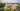The average SAT score of 1061 breaks down into:

• SAT math: 510